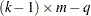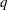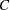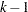MODEL Statement

<label:> MODEL response=effects </ options> ;
<label:> MODEL events/trials=effects </ options> ;

The MODEL statement names the variables used as the response and the independent variables. Additionally, you can specify the distribution used to model the response, as well as other options. Only a single MODEL statement can be used with one invocation of the PROBIT procedure. If multiple MODEL statements are present, only the last is used. Main effects and interaction terms can be specified in the MODEL statement, as in the GLM procedure.

The optional label, which must be a valid SAS name, is used to label output from the matching MODEL statement.

The response can be a single variable with a value that is used to indicate the level of the observed response. For example, the response might be a variable called Symptoms that takes on the values ‘None,’ ‘Mild,’ or ‘Severe.’ Note that, for dichotomous response variables, the probability of the lower sorted value is modeled by default (see the section Details: PROBIT Procedure). Because the model fit by the PROBIT procedure requires ordered response levels, you might need to use either the ORDER=DATA option in the PROC PROBIT statement or a numeric coding of the response to get the desired ordering of levels.

Alternatively, the response can be specified as a pair of variable names separated by a slash (/). The value of the first variable, events, is the number of positive responses (or events). The value of the second variable, trials, is the number of trials. Both variables must be numeric and nonnegative, and the ratio of the first variable value to the second variable value must be between 0 and 1, inclusive. For example, the variables might be hits, a variable containing the number of hits for a baseball player, and AtBats, a variable containing the number of times at bat. A model for hitting proportion (batting average) as a function of age could be specified as

```model hits/AtBats=age;
```

The effects following the equal sign are the covariates in the model. Higher-order effects, such as interactions and nested terms, are allowed in the list, as in the GLM procedure. Variable names and combinations of variable names representing higher-order terms are allowed to appear in this list. Classification variables can be used as effects, and indicator variables are generated for the class levels. If you do not specify any covariates following the equal sign, an intercept-only model is fit.

The following options are available in the MODEL statement.

AGGREGATE
AGGREGATE=variable-list

specifies the subpopulations on which the Pearson’s chi-square test statistic and the log-likelihood ratio chi-square test statistic (deviance) are calculated if the LACKFIT option is specified. See the section Rescaling the Covariance Matrix for details of Pearson’s chi-square and deviance calculations.

Observations with common values in the given list of variables are regarded as coming from the same subpopulation. Variables in the list can be any variables in the input data set. Specifying the AGGREGATE option is equivalent to specifying the AGGREGATE= option with a variable list that includes all independent variables in the MODEL statement. The PROBIT procedure sorts the input data set according to the variables specified in this list. Information for the sorted data set is reported in the "Response-Covariate Profile" table.

The deviance and Pearson’s goodness-of-fit statistics are calculated if the LACKFIT option is specified in the MODEL statement. The calculated results are reported in the "Goodness-of-Fit" table. If the Pearson’s chi-square test is significant with the test level specified by the HPROB= option, the fiducial limits, if required with the INVERSECL option in the MODEL statement, are modified (see the section Inverse Confidence Limits for details). Also, the covariance matrix is rescaled by the dispersion parameter when the SCALE= option is specified.

ALPHA=value

sets the significance level for the confidence intervals for regression parameters, fiducial limits for the predicted values, and confidence intervals for the predicted probabilities. The value must be between 0 and 1. The default value is ALPHA=0.05.

CONVERGE=value

specifies the convergence criterion. Convergence is declared when the maximum change in the parameter estimates between Newton-Raphson steps is less than the value specified. The change is a relative change if the parameter is greater than 0.01 in absolute value; otherwise, it is an absolute change.

By default, CONVERGE=1.0E8.

CORRB

displays the estimated correlation matrix of the parameter estimates.

COVB

displays the estimated covariance matrix of the parameter estimates.

DISTRIBUTION=distribution-type
DIST=distribution-type
D=distribution-type

specifies the cumulative distribution function used to model the response probabilities. The distributions are described in the section Details: PROBIT Procedure. Valid values for distribution-type are as follows:

NORMAL

the normal distribution for the probit model

LOGISTIC

the logistic distribution for the logit model

EXTREMEVALUE | EXTREME | GOMPERTZ

the extreme value, or Gompertz distribution for the gompit model

By default, DISTRIBUTION=NORMAL.

HPROB=p

specifies a minimum probability level for the Pearson’s chi-square to indicate a good fit. The default value is 0.10. The LACKFIT option must also be specified for this option to have any effect. For Pearson’s goodness-of-fit chi-square values with probability greater than the HPROB= value, the fiducial limits, if requested with the INVERSECL option, are computed by using a critical value of 1.96. For chi-square values with probability less than the value of the HPROB= option, the critical value is a 0.95 two-sided quantile value taken from thedistribution with degrees of freedom equal to, whereis the number of levels for the response variable,is the number of different sets of independent variable values, andis the number of parameters fit in the model. If you specify the HPROB= option in both the PROC PROBIT and MODEL statements, the MODEL statement option takes precedence.

INITIAL=values

sets initial values for the parameters in the model other than the intercept. The values must be given in the order in which the variables are listed in the MODEL statement. If some of the independent variables listed in the MODEL statement are classification variables, then there must be as many values given for that variable as there are classification levels minus 1. The INITIAL option can be specified as follows.

 Type of List Specification List separated by blanks initial=3 4 5 List separated by commas initial=3,4,5

By default, all parameters have initial estimates of zero.

Note: The INITIAL= option is overwritten by the INEST= option in the PROC PROBIT statement.

INTERCEPT=value

initializes the intercept parameter to value. By default, INTERCEPT=0.

INVERSECL<(PROB=rates)>

computes confidence limits for the values of the first continuous independent variable (such as dose) that yield selected response rates. You can optionally specify a list of response rates as rates. The response rates must be between zero and one; they can be a list separated by blanks, commas, or in the form of a DO list. For example, the following expressions are all valid lists of response rates:

```PROB = .1 TO .9 by .1
PROB = .1 .2 .3 .4
PROB = .01, .25, .75, .9
```

If the algorithm fails to converge (this can happen whenis nonzero), missing values are reported for the confidence limits. See the section Inverse Confidence Limits for details.

ITPRINT

displays the iteration history, the final evaluation of the gradient, and the second derivative matrix (Hessian).

LACKFIT

performs two goodness-of-fit tests (a Pearson’s chi-square test and a log-likelihood ratio chi-square test) for the fitted model.

To compute the test statistics, proper grouping of the observations into subpopulations is needed. You can use the AGGREGATE or AGGREGATE= option for this purpose. See the entry for the AGGREGATE and AGGREGATE= options under the MODEL statement. If neither AGGREGATE nor AGGREGATE= is specified, PROC PROBIT assumes each observation is from a separate subpopulation and computes the goodness-of-fit test statistics only for the events/trials syntax.

Note: This test is not appropriate if the data are very sparse, with only a few values at each set of the independent variable values.

If the Pearson’s chi-square test statistic is significant, then the covariance estimates and standard error estimates are adjusted. See the section Lack-of-Fit Tests for a description of the tests. Note that the LACKFIT option can also appear in the PROC PROBIT statement. See the section PROC PROBIT Statement for details.

MAXITER=value
MAXIT=value

specifies the maximum number of iterations to be performed in estimating the parameters. By default, MAXITER=50.

NOINT

fits a model with no intercept parameter. If the INTERCEPT= option is also specified, the intercept is fixed at the specified value; otherwise, it is set to zero. This is most useful when the response is binary. When the response haslevels, thenintercept parameters are fit. The NOINT option sets the intercept parameter corresponding to the lowest response level equal to zero. A Lagrange multiplier, or score, test for the restricted model is computed when the NOINT option is specified.

SCALE=scale

enables you to specify the method for estimating the dispersion parameter. To correct for overdispersion or underdispersion, the covariance matrix is multiplied by the estimate of the dispersion parameter. Valid values for scale are as follows:

D  |  DEVIANCE

specifies that the dispersion parameter be estimated by the deviance divided by its degrees of freedom.

P  |  PEARSON

specifies that the dispersion parameter be estimated by the Pearson’s chi-square statistic divided by its degrees of freedom. This is set as the default method for estimating the dispersion parameter.

You can use the AGGREGATE= option to define the subpopulations for calculating the Pearson’s chi-square statistic and the deviance.

The "Goodness-of-Fit " table includes the Pearson’s chi-square statistic, the deviance, their degrees of freedom, the ratio of each statistic divided by its degrees of freedom, and the corresponding-value.

SINGULAR=value

specifies the singularity criterion for determining linear dependencies in the set of independent variables. The sum of squares and crossproducts matrix of the independent variables is formed and swept. If the relative size of a pivot becomes less than the value specified, then the variable corresponding to the pivot is considered to be linearly dependent on the previous set of variables considered. By default, value=1E12.